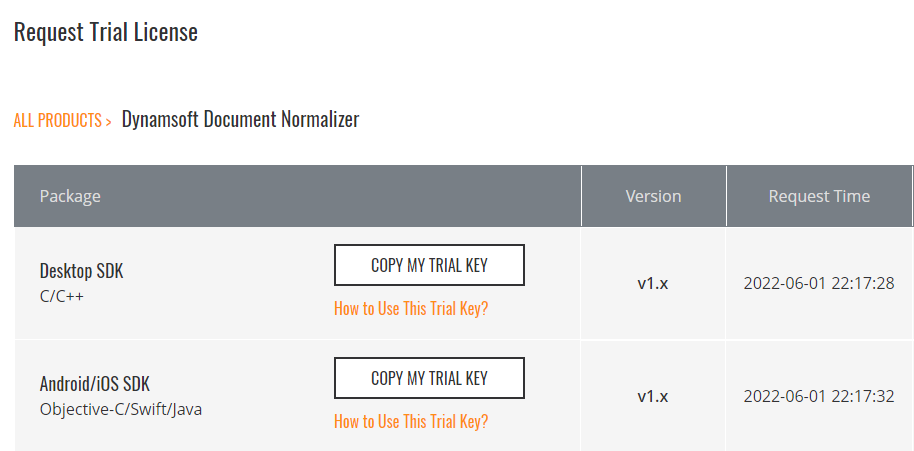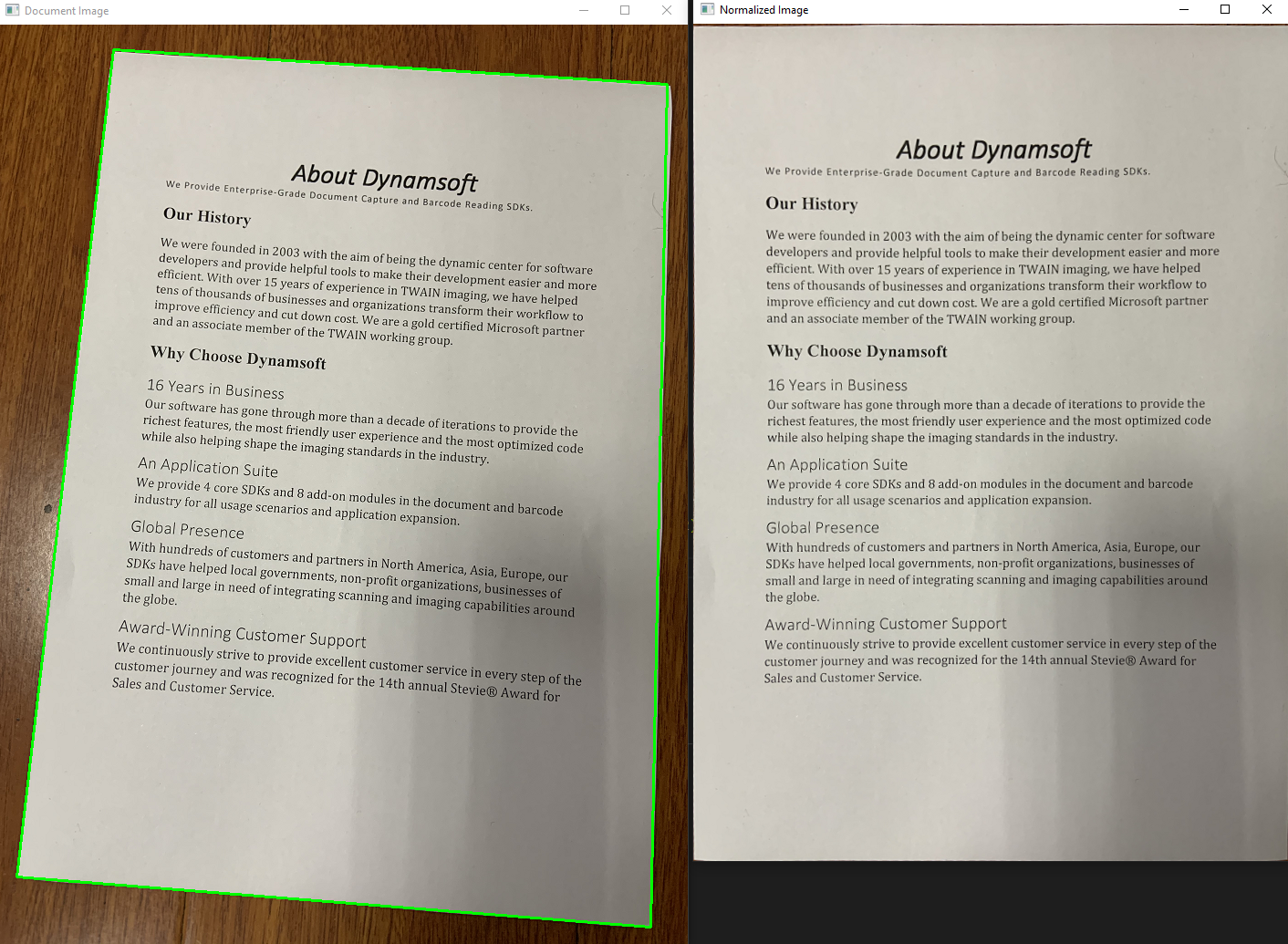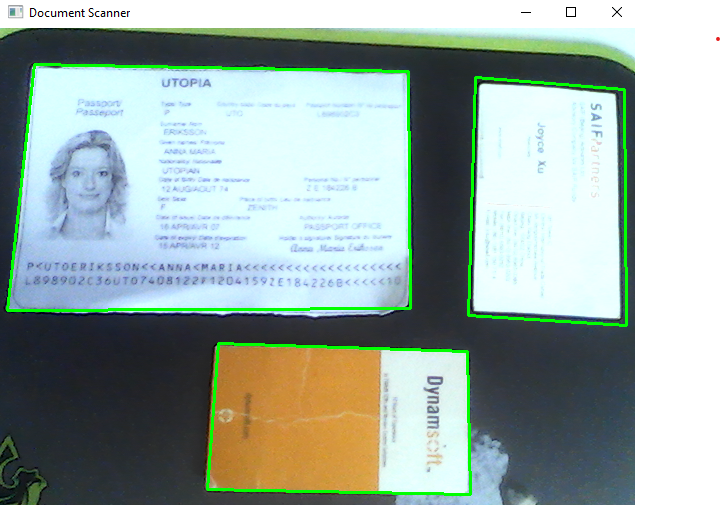×

# How to Implement Python Document Scanner on Windows and Linux

Dynamsoft Document Normalizer is a document scanning SDK that can be used to do edge detection, perspective correction, and contrast enhancement. Its C/C++ API is available on Windows and Linux. In this article, we will show you how to build a Python wrapper for Dynamsoft C/C++ Document Normalizer SDK, as well as how to quickly implement a document scanner with Python on Windows and Linux.

## Python Wrapper for Dynamsoft C/C++ Document Normalizer SDK

Here we are not going to talk about how to build a Python C/C++ extension from scratch. If you are interested in this topic, you can refer to this article. Instead, we will focus on the C/C++ implementation of the Python wrapper for Dynamsoft C/C++ Document Normalizer SDK.

### Customized PyObjects in C/C++

We define three native `PyObject`s, which will be interacted with Python code:

• DynamsoftDocumentScanner: the native object that holds the pointer to the C/C++ Document Normalizer instance
``````  typedef struct
{
void *handler;
PyObject *callback;
} DynamsoftDocumentScanner;
``````
• DocumentResult: the native object that holds the contour points of the detected document
``````  typedef struct
{
PyObject *confidence;
PyObject *x1;
PyObject *y1;
PyObject *x2;
PyObject *y2;
PyObject *x3;
PyObject *y3;
PyObject *x4;
PyObject *y4;
} DocumentResult;
``````
• NormalizedImage: the native object that holds the normalized image buffer
``````  typedef struct
{
PyObject *bytearray;
PyObject *length;
PyObject *width;
PyObject *height;
PyObject *stride;
PyObject *format;
NormalizedImageResult* normalizedResult;
} NormalizedImage;
``````

### Methods Implementation

The `DynamsoftDocumentScanner` module contains following methods:

``````static PyMethodDef instance_methods[] = {
{"detectFile", detectFile, METH_VARARGS, NULL},
{"detectMat", detectMat, METH_VARARGS, NULL},
{"detectMatAsync", detectMatAsync, METH_VARARGS, NULL},
{"setParameters", setParameters, METH_VARARGS, NULL},
{"normalizeFile", normalizeFile, METH_VARARGS, NULL},
{"normalizeBuffer", normalizeBuffer, METH_VARARGS, NULL},
{NULL, NULL, 0, NULL}
};
``````
• `detectFile`: Detects the document in the image file and returns the contour points.
``````  static PyObject *detectFile(PyObject *obj, PyObject *args)
{
DynamsoftDocumentScanner *self = (DynamsoftDocumentScanner *)obj;

char *pFileName; // File name
if (!PyArg_ParseTuple(args, "s", &pFileName))
{
return NULL;
}

int ret = DDN_DetectQuadFromFile(self->handler, pFileName, "", &pResults);
if (ret)
{
printf("Detection error: %s\n", DC_GetErrorString(ret));
}

PyObject *list = createPyList(pResults);

// Release memory
if (pResults != NULL)

return list;
}
``````
• `detectMat`: Detects the document in the image buffer and returns the contour points.
``````  static PyObject *detectMat(PyObject *obj, PyObject *args)
{
DynamsoftDocumentScanner *self = (DynamsoftDocumentScanner *)obj;

PyObject *o;
if (!PyArg_ParseTuple(args, "O", &o))
return NULL;

Py_buffer *view;
int nd;
PyObject *memoryview = PyMemoryView_FromObject(o);
if (memoryview == NULL)
{
PyErr_Clear();
return NULL;
}

view = PyMemoryView_GET_BUFFER(memoryview);
char *buffer = (char *)view->buf;
nd = view->ndim;
int len = view->len;
int stride = view->strides;
int width = view->strides / view->strides;
int height = len / stride;

ImagePixelFormat format = IPF_RGB_888;

if (width == stride)
{
format = IPF_GRAYSCALED;
}
else if (width * 3 == stride)
{
format = IPF_RGB_888;
}
else if (width * 4 == stride)
{
format = IPF_ARGB_8888;
}

ImageData data;
data.bytes = (unsigned char *)buffer;
data.width = width;
data.height = height;
data.stride = stride;
data.format = format;
data.bytesLength = len;

int ret = DDN_DetectQuadFromBuffer(self->handler, &data, "", &pResults);
if (ret)
{
printf("Detection error: %s\n", DC_GetErrorString(ret));
}

PyObject *list = createPyList(pResults);

// Release memory
if (pResults != NULL)

Py_DECREF(memoryview);

return list;
}
``````
• `addAsyncListener` and `detectMatAsync`: Uses a native thread to run edge detection task asynchronously and returns the contour points.
``````  static PyObject *addAsyncListener(PyObject *obj, PyObject *args)
{
DynamsoftDocumentScanner *self = (DynamsoftDocumentScanner *)obj;

PyObject *callback = NULL;
if (!PyArg_ParseTuple(args, "O", &callback))
{
return NULL;
}

if (!PyCallable_Check(callback))
{
PyErr_SetString(PyExc_TypeError, "parameter must be callable");
return NULL;
}
else
{
Py_XINCREF(callback);       /* Add a reference to new callback */
Py_XDECREF(self->callback); /* Dispose of previous callback */
self->callback = callback;
}

if (self->worker == NULL)
{
self->worker->running = true;
}

return Py_BuildValue("i", 0);
}

static PyObject *detectMatAsync(PyObject *obj, PyObject *args)
{
DynamsoftDocumentScanner *self = (DynamsoftDocumentScanner *)obj;
PyObject *o;
if (!PyArg_ParseTuple(args, "O", &o))
return NULL;

Py_buffer *view;
int nd;
PyObject *memoryview = PyMemoryView_FromObject(o);
if (memoryview == NULL)
{
PyErr_Clear();
return NULL;
}

view = PyMemoryView_GET_BUFFER(memoryview);
char *buffer = (char *)view->buf;
nd = view->ndim;
int len = view->len;
int stride = view->strides;
int width = view->strides / view->strides;
int height = len / stride;

ImagePixelFormat format = IPF_RGB_888;

if (width == stride)
{
format = IPF_GRAYSCALED;
}
else if (width * 3 == stride)
{
format = IPF_RGB_888;
}
else if (width * 4 == stride)
{
format = IPF_ARGB_8888;
}

unsigned char *data = (unsigned char *)malloc(len);
memcpy(data, buffer, len);

std::unique_lock<std::mutex> lk(self->worker->m);
{
std::queue<std::function<void()>> empty = {};
}
std::function<void()> task_function = std::bind(scan, self, data, width, height, stride, format, len);
self->worker->cv.notify_one();
lk.unlock();

Py_DECREF(memoryview);
return Py_BuildValue("i", 0);
}
``````
• `setParameters`: Sets the parameters for the document scanner SDK.
``````  static PyObject *setParameters(PyObject *obj, PyObject *args)
{
DynamsoftDocumentScanner *self = (DynamsoftDocumentScanner *)obj;

const char*params;
if (!PyArg_ParseTuple(args, "s", &params))
{
return NULL;
}

char errorMsgBuffer;
int ret = DDN_InitRuntimeSettingsFromString(self->handler, params, errorMsgBuffer, 512);
printf("Init runtime settings: %s\n", errorMsgBuffer);

return Py_BuildValue("i", ret);
}
``````
• `normalizeFile`: Normalizes the document based on the contour points.
``````  static PyObject *normalizeFile(PyObject *obj, PyObject *args)
{
DynamsoftDocumentScanner *self = (DynamsoftDocumentScanner *)obj;

char *pFileName;
int x1, y1, x2, y2, x3, y3, x4, y4;
if (!PyArg_ParseTuple(args, "siiiiiiii", &pFileName, &x1, &y1, &x2, &y2, &x3, &y3, &x4, &y4))
return NULL;

NormalizedImageResult* normalizedResult = NULL;

int errorCode = DDN_NormalizeFile(self->handler, pFileName, "", &quad, &normalizedResult);
if (errorCode != DM_OK)
printf("%s\r\n", DC_GetErrorString(errorCode));

PyObject *normalizedImage = createNormalizedImage(normalizedResult);

return normalizedImage;
}
``````
• `normalizeBuffer`: Normalizes the document based on the contour points.
``````  static PyObject *normalizeBuffer(PyObject *obj, PyObject *args)
{
DynamsoftDocumentScanner *self = (DynamsoftDocumentScanner *)obj;

PyObject *o;
int x1, y1, x2, y2, x3, y3, x4, y4;
if (!PyArg_ParseTuple(args, "Oiiiiiiii", &o, &x1, &y1, &x2, &y2, &x3, &y3, &x4, &y4))
return NULL;

Py_buffer *view;
int nd;
PyObject *memoryview = PyMemoryView_FromObject(o);
if (memoryview == NULL)
{
PyErr_Clear();
return NULL;
}

view = PyMemoryView_GET_BUFFER(memoryview);
char *buffer = (char *)view->buf;
nd = view->ndim;
int len = view->len;
int stride = view->strides;
int width = view->strides / view->strides;
int height = len / stride;

ImagePixelFormat format = IPF_RGB_888;

if (width == stride)
{
format = IPF_GRAYSCALED;
}
else if (width * 3 == stride)
{
format = IPF_RGB_888;
}
else if (width * 4 == stride)
{
format = IPF_ARGB_8888;
}

ImageData data;
data.bytes = (unsigned char *)buffer;
data.width = width;
data.height = height;
data.stride = stride;
data.format = format;
data.bytesLength = len;

NormalizedImageResult* normalizedResult = NULL;
int errorCode = DDN_NormalizeBuffer(self->handler, &data, "", &quad, &normalizedResult);
if (errorCode != DM_OK)
printf("%s\r\n", DC_GetErrorString(errorCode));

PyObject *normalizedImage = createNormalizedImage(normalizedResult);

Py_DECREF(memoryview);

return normalizedImage;
}
``````

The `NormalizedImage` module contains two methods: `save()` and `recycle()`. Since the object holds the pointer of the native image data, the `recycle()` method must be called when the `NormalizedImage` object is no more used.

``````static PyMethodDef ni_instance_methods[] = {
{"save", save, METH_VARARGS, NULL},
{"recycle", recycle, METH_VARARGS, NULL},
{NULL, NULL, 0, NULL}
};

static PyObject *save(PyObject *obj, PyObject *args)
{
NormalizedImage *self = (NormalizedImage *)obj;

char *pFileName; // File name
if (!PyArg_ParseTuple(args, "s", &pFileName))
{
return NULL;
}

if (self->normalizedResult)
{
DDN_SaveImageDataToFile(self->normalizedResult->image, pFileName);
printf("Save image to file: %s\n", pFileName);
return Py_BuildValue("i", 0);
}

return Py_BuildValue("i", -1);
}

static PyObject *recycle(PyObject *obj, PyObject *args)
{
NormalizedImage *self = (NormalizedImage *)obj;

if (self->normalizedResult)
{
DDN_FreeNormalizedImageResult(&self->normalizedResult);
self->normalizedResult = NULL;
}

return Py_BuildValue("i", 0);
}
``````

The `document-scanner-sdk` package is available on https://pypi.org/project/document-scanner-sdk/.

## Get a 30-Day Free Trial License

To activate the SDK, you need to get a 30-day free trial license from the online portal.## Python Document Scanner

Install OpenCV and Document Scanner SDK:

``````pip install opencv-python document-scanner-sdk
``````

The OpenCV package depends on `NumPy`, which will be installed along with it.

### Scan Documents from Image Files

Let’s get started with a simple example. The following code snippet shows how to use the SDK to scan a document from an image file.

``````from time import sleep
import docscanner
import numpy as np
import cv2
import time

def showNormalizedImage(name, normalized_image):
mat = docscanner.convertNormalizedImage2Mat(normalized_image)
cv2.imshow(name, mat)
return mat

scanner = docscanner.createInstance()

ret = scanner.setParameters(docscanner.Templates.color)
results = scanner.detectMat(image)
for result in results:
x1 = result.x1
y1 = result.y1
x2 = result.x2
y2 = result.y2
x3 = result.x3
y3 = result.y3
x4 = result.x4
y4 = result.y4

normalized_image = scanner.normalizeBuffer(image, x1, y1, x2, y2, x3, y3, x4, y4)
normalized_image.recycle()

showNormalizedImage("Normalized Image", normalized_image)
cv2.drawContours(image, [np.int0([(x1, y1), (x2, y2), (x3, y3), (x4, y4)])], 0, (0, 255, 0), 2)

cv2.imshow('Document Image', image)
cv2.waitKey(0)
``````### Scan Documents from Camera

Now, we move to the camera example with detailed steps.

We utilize OpenCV’s video I/O API to capture and show camera video stream:

``````import cv2
cap = cv2.VideoCapture(0)
while True:

ch = cv2.waitKey(1)
if ch == 27:
break

cv2.imshow('Document Scanner', image)
``````

Initialize the document scanner with a valid license key:

``````import docscanner
scanner = docscanner.createInstance()
``````

You can select a built-in template to set the output type of a normalized document: `binary`, `grayscale`, or `color`.

``````scanner.setParameters(docscanner.Templates.color)
``````

The template can be customized by referring to the template documentation. A more complicated parameter template looks like this:

``````{
"GlobalParameter":{
"Name":"GP",
"MaxTotalImageDimension":0
},
"ImageParameterArray":[
{
"Name":"IP-1",
"RegionPredetectionModes":[
{
"Mode": "RPM_GENERAL"
}
],
"Timeout": 10000,
"ColourChannelUsageType": "CCUT_AUTO",
"ScaleDownThreshold": 2300,
"ColourConversionModes":[
{
"Mode": "CICM_GENERAL",
"BlueChannelWeight": -1,
"GreenChannelWeight": -1,
"RedChannelWeight": -1
}
],
"GrayscaleTransformationModes":[
{
"Mode": "GTM_ORIGINAL"
}
],
"GrayscaleEnhancementModes": [
{
"Mode": "GEM_GENERAL"
}
],
"BinarizationModes":[
{
"Mode": "BM_LOCAL_BLOCK",
"BlockSizeX": 0,
"BlockSizeY": 0,
"EnableFillBinaryVacancy": 0,
"MorphOperation": "Close",
"MorphShape": "Rectangle",
"MorphOperationKernelSizeX": -1,
"MorphOperationKernelSizeY": -1,
"ThresholdCompensation": 10
}
],
"TextureDetectionModes":[
{
"Mode": "TDM_GENERAL_WIDTH_CONCENTRATION",
"Sensitivity": 5
}
],
"TextFilterModes": [
{
"Mode": "TFM_GENERAL_CONTOUR",
"IfEraseTextZone": 0,
"MinImageDimension": 65536,
"Sensitivity": 0
}
],
"LineExtractionModes": [
{
"Mode": "LEM_GENERAL"
}
],
"NormalizerParameterName":"NP-1",
"BaseImageParameterName":""
}
],
"NormalizerParameterArray":[
{
"Name":"NP-1",
"ContentType": "CT_DOCUMENT",
"InteriorAngleRangeArray": [
{
"MinValue": 70,
"MaxValue": 110
}
],
{
"Mode": "QDM_GENERAL"
}
],
"DeskewMode": {
"Mode": "DM_PERSPECTIVE_CORRECTION",
"ContentDirection": 0
},
"PageSize": [-1, -1],
"ColourMode": "ICM_BINARY",
"Brightness": 0,
"Contrast": 0
}
]
}
``````

In live camera scenarios, all CPU-intensive tasks, such as document edge detection, should be executed in a separate thread to avoid blocking the main thread. Therefore, we register a callback function to a native thread and call the asynchronous detection method to queue the detection tasks that will be consumed by the native thread:

``````g_results = None
g_normalized_images = []
index = 0

def callback(results):
global g_results
g_results = results

def process_video(scanner):
global g_normalized_images, index

cap = cv2.VideoCapture(0)
while True:

ch = cv2.waitKey(1)
if ch == 27:
break
if image is not None:
scanner.detectMatAsync(image)

if g_results != None:
for result in g_results:
x1 = result.x1
y1 = result.y1
x2 = result.x2
y2 = result.y2
x3 = result.x3
y3 = result.y3
x4 = result.x4
y4 = result.y4

cv2.drawContours(image, [np.int0([(x1, y1), (x2, y2), (x3, y3), (x4, y4)])], 0, (0, 255, 0), 2)

cv2.imshow('Document Scanner', image)
``````

So far, the real-time document edge detection function has been implemented. To crop the document based on the contour points and take a further step to normalize the document, we need to call the `normalizeBuffer` method:

``````def showNormalizedImage(name, normalized_image):
mat = docscanner.convertNormalizedImage2Mat(normalized_image)
cv2.imshow(name, mat)
return mat

if ch == ord('n'): # normalize image
if g_results != None:

if len(g_results) > 0:
for result in g_results:
x1 = result.x1
y1 = result.y1
x2 = result.x2
y2 = result.y2
x3 = result.x3
y3 = result.y3
x4 = result.x4
y4 = result.y4

normalized_image = scanner.normalizeBuffer(image, x1, y1, x2, y2, x3, y3, x4, y4)
g_normalized_images.append((str(index), normalized_image))
showNormalizedImage(str(index), normalized_image)
index += 1
else:
print('No document found')
``````

If you are satisfied with the result, you can save the normalized image to a file:

``````if ch == ord('s'): # save image
if len(g_normalized_images) > 0:
for data in g_normalized_images:
cv2.destroyWindow(data)
data.save(str(time.time()) + '.png')
print('Image saved')
data.recycle()

g_normalized_images = []
index = 0
``````

Finally, let’s run the Python document scanner app in the terminal.## Source Code

https://github.com/yushulx/python-document-scanner-sdk